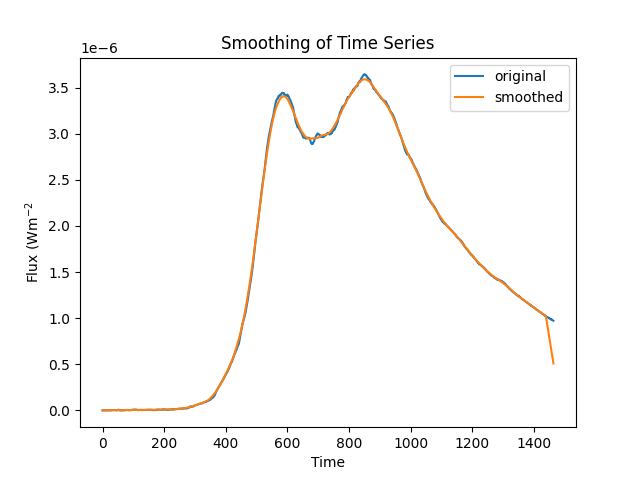# Smoothing of timeSeries data using convolution filters¶

How to smooth a TimeSeries using a convolution filter kernel from `convolution` and `convolve` function.

```import matplotlib.pyplot as plt
from astropy.convolution import convolve, Box1DKernel

from sunpy.timeseries import TimeSeries
from sunpy.data.sample import NOAAINDICES_TIMESERIES as noaa_ind
```

Let’s first create a TimeSeries from sample data

```ts_noaa_ind = TimeSeries(noaa_ind, source='NOAAIndices')
```

Now we will extract data values from the TimeSeries and apply a BoxCar filter to get smooth data. Boxcar smoothing is equivalent to taking our signal and using it to make a new signal where each element is the average of w adjacent elements. Here we will use AstroPy’s convolve function with a “boxcar” kernel of width w = 10.

```ts_noaa_ind.data['sunspot SWO Smoothed'] = convolve(
ts_noaa_ind.data['sunspot SWO'].values, kernel=Box1DKernel(10))
```

Plotting original and smoothed timeseries

```plt.ylabel('Sunspot Number')
plt.xlabel('Time')
plt.title('Smoothing of Time Series')
plt.plot(ts_noaa_ind.data['sunspot SWO'])
plt.plot(ts_noaa_ind.data['sunspot SWO Smoothed'])
plt.legend()
plt.show()
```Out:

```No handles with labels found to put in legend.
```

Total running time of the script: ( 0 minutes 0.340 seconds)

Gallery generated by Sphinx-Gallery